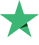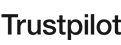watching now
5 Students
118 Lessons
Intermediate

#### What Will You Learn?

• Learn Machine Learning Models in R Programming Language
• Learn the Intuition of each Model
• Learn to choose the best Machine Learning Model for a specific problem
• Learn the Math behind every Machine Learning Model
• Learn to make simple and GUI Based Templates

#### Curriculum

16h 16m
10:24
##### Section 2: Simple Linear Regression Statistics
36:13
Simple Linear Regression Statistics 2
05:56
Simple Linear Regression Statistics 3
20:40
##### Section 3: Simple Linear Regression in R
51:53
Simple Linear Regression in R Part-1
08:31
Simple Linear Regression in R Part-2
07:20
Simple Linear Regression in R Part-3
09:49
Simple Linear Regression in R Part-4
02:45
Simple Linear Regression in R Part-5
05:13
Simple Linear Regression in R Part-6
18:15
##### Section 4: Multiple Linear Regression Statistics
56:48
Multiple Linear Regression Statistics 1
09:22
Multiple Linear Regression Statistics 2
05:51
Multiple Linear Regression Statistics 3
10:38
Multiple Linear Regression Statistics 4
13:30
Multiple Linear Regression Statistics 5
07:21
Multiple Linear Regression Statistics 6
08:57
Data set for Multiple Linear Regression Model
01:09
##### Section 5: Polynomial Regression Statistics
07:12
Polynomial Regression Statistics 1
07:12
##### Section 6: Polynomial Regression in R
31:49
Polynomial Regression in R Part-1
03:12
Polynomial Regression in R Part-2
09:54
Polynomial Regression in R Part-3
05:13
Polynomial Regression in R Part-4
06:22
Polynomial Regression in R Part-5
07:08
##### Section 7: Ridge Regression Statistics
16:09
Ridge Regression Statistics 1
04:52
Ridge Regression Statistics 2
11:17
##### Section 8: Ridge Regression in R
54:52
Ridge Regression in R Part-1
17:10
Ridge Regression in R Part-2
25:58
Ridge Regression in R Part-3
04:12
Ridge Regression in R Part-4
07:32
##### Section 9: Lasso Regression Statistic
08:57
Lasso Regression Statistic 1
08:57
##### Section 10: Lasso Regression in R
46:08
Lasso Regression in R Part-1
14:24
Lasso Regression in R Part-2
19:48
Lasso Regression in R Part-3
04:36
Lasso Regression in R Part-4
07:20
##### Section 11: Elastic Net Regression Statistics
02:33
Elastic Net Regression Statistics 1
02:33
##### Section 12: Elastic Net Regression in R
50:37
Elastic Net Regression in R Part-1
16:21
Elastic Net Regression in R Part-2
21:35
Elastic Net Regression in R Part-3
05:14
Elastic Net Regression in R Part-4
07:27
##### Section 13: Decision Tree Regression in R
41:53
Decision Tree Regression in R Part-1
08:23
Decision Tree Regression in R Part-2
07:05
Decision Tree Regression in R Part-3
07:24
Decision Tree Regression in R Part-4
04:58
Decision Tree Regression in R Part-5
14:03
##### Section 14: Decision Tree Regression Statistics
06:54
Decision Tree Regression Statistics 1
06:54
##### Section 15: Random Forest Regression Statistics
09:47
Random Forest Regression Statistics 1
09:47
##### Section 16: Random Forest Regression in R
38:02
Random Forest Regression in R Part-1
07:07
Random Forest Regression in R Part-2
04:09
Random Forest Regression in R Part-3
08:08
Random Forest Regression in R Part-4
04:55
Random Forest Regression in R Part-5
13:43
##### Section 17: +++++++++Beginning Classification+++++++++
12:06
Confusion Matrix
07:05
Sparse Matrix
03:37
Data set for Classification Models
01:24
##### Section 18: Logistic Regression Statistics
12:23
Logistic Regression Statistics 1
12:23
##### Section 19: Logistic Regression in R
51:13
Logistic Regression in R Part-1
03:19
Logistic Regression in R Part-2
08:49
Logistic Regression in R Part-3
03:19
Logistic Regression in R Part-4
03:56
Logistic Regression in R Part-5
16:22
Logistic Regression in R Part-6
15:28
##### Section 20: Support Vector Classification
08:12
Support Vector Classification Statistics 1
08:12
##### Section 21: Support Vector Classification in R
21:47
Support Vector Classification in R Part-1
11:12
Support Vector Classification in R Part-2
02:34
Support Vector Classification in R Part-3
08:01
06:05
KNN Statistics
06:05
20:53
KNN in R Part-1
15:52
KNN in R Part-2
05:01
##### Section 24: Naive Bayes Classification Model Intuition
19:00
Naive Bayes Intuition 1
08:52
Naive Bayes Intuition 1
10:08
##### Section 25: Naive Bayes Classification Model in R
38:52
Naive Bayes in R Part-1
10:04
Naive Bayes in R Part-2
02:32
Naive Bayes in R Part-3
12:17
Naive Bayes in R Part-4
13:59
##### Section 26: Decision Tree Classification Statistics
20:06
Decision Tree Classification Statistics 1
08:23
Decision Tree Classification Statistics 2
11:43
##### Section 27: Decision Tree Classification in R
45:46
Decision Tree Classification in R Part-1
09:42
Decision Tree Classification in R Part-2
04:28
Decision Tree Classification in R Part-3
02:38
Decision Tree Classification in R Part-4
12:21
Decision Tree Classification in R Part-5
16:37
##### Section 28: Random Forest Classification Statistics
10:40
Random Forest Classification Statistics 1
10:40
##### Section 29: Random Forest Classification in R
24:57
Random Forest Classification in R Part 1
14:06
Random Forest Classification in R Part 2
02:17
Random Forest Classification in R Part 3
08:34
##### Section 30: Beginning Clustering
08:47
What is Clustering
06:57
Data set for Clustering
01:50
##### Section 31: K Means Clustering Statistics
15:01
K Means Clustering Statistics 1
09:13
K Means Clustering Statistics 2
05:48
##### Section 32: K Means Clustering in R
25:58
K Means Clustering in R Part-1
05:47
K Means Clustering in R Part-2
11:58
K Means Clustering in R Part-3
08:13
##### Section 33: Hierarchical Clustering
44:10
Hierarchical Clustering Statistics 1
08:04
Hierarchical Clustering Statistics 2
25:16
Hierarchical Clustering Statistics 3
10:50
##### Section 34: Hierarchical Clustering in R
18:09
Hierarchical Clustering in R Part 1
09:21
Hierarchical Clustering in R Part 2
08:48
##### Section 35: Apriori Algorithm Statistics
13:37
Apriori Algorithm Statistic 1
13:37
##### Section 36: Apriori Algorithm in R
20:29
Apriori Algorithm in R Part-1
08:20
Apriori Algorithm in R Part-2
02:07
Apriori Algorithm in R Part-3
10:02
##### Section 37: Eclat Algorithm Statistics
06:18
Eclat Algorithm Statistic 1
06:18
##### Section 38: Eclat Algorithm in R
14:05
Eclat Algorithm in R Part 1
08:12
Eclat Algorithm in R Part 2
05:53
##### Section 39: Starting Natural Language Processing
47:27
Understanding Natural Language Processing
09:17
Natural Language Processing in R Part 1
04:23
Natural Language Processing in R Part 2
05:46
Natural Language Processing in R Part 3
03:10
Natural Language Processing in R Part 4
03:04
Natural Language Processing in R Part 5
02:48
Natural Language Processing in R Part 6
02:00
Natural Language Processing in R Part 7
01:00
Natural Language Processing in R Part 8
02:39
Natural Language Processing in R Part 9
12:20
Natural Language Processing in R Part 10
01:00

#### Requirements

• Basics of any programming language required

#### Coding School

100% of students rated this instructor as excellent!
Reviews 1
Students 14
Courses 6

I am a Software Engineer, Data Scientist and Entrepreneur. I started coding when i was only 12. I love teaching to people who are curious to learn. I think BitDegree is the platform which is going to be the hub of online education in the future so that is why i am here to help you guys who are curious to learn

I love to teach the newbies and also the professionals. I always try to teach something new and in-demand topics to my students. I teach with passion and purpose! Every course is delivered with my students in mind. I am here to change your Life!

#### BitDegree platform reviews

Our students say Excellent
4.5 out of 5